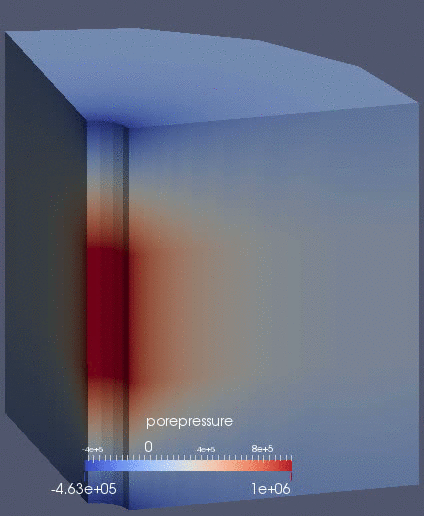# Porous Flow Tutorial Page 01. A single fluid

This tutorial page describes how PorousFlow can be used to solve a very simple fluid-flow problem. The physics are described by the equation (1) This is the simplest type of equation that PorousFlow solves. It is often used in thermo-poro-elasticity, but PorousFlow is often employed to solve much more complex equations. See governing equations for further details, and PorousFlowFullySaturatedMassTimeDerivative and PorousFlowFullySaturatedDarcyBase for the derivation of Eq. (1) from the general governing equations. In this equation:

• is the fluid porepressure (units of Pa)

• an over-dot represents a time derivative

• is the Biot Modulus (units of Pa)

• is the Biot coefficient (dimensionless)

• is the rate of volumetric strain of the solid rock (units s)

• is the effective volumetric thermal expansion coefficient (units K)

• is the temperature (units K)

• represents a spatial derivative

• is the permeability tensor (units m)

• is the fluid viscosity (units Pa.s)

• is the fluid density (units kg.m)

• is the gravitational acceleration (units m.s)

The units suggested here are not manditory: any consistent unit system may be used in MOOSE. For instance, in reservoir physics it is often convenient to express everything in MPa and years rather than Pa and seconds.

The Biot modulus is (2) and the effective volumetric thermal expansion coefficient is (3) In these equations

• is the porosity (dimensionless)

• is the bulk modulus of the fluid (units Pa)

• is the bulk modulus of the drained porous skeleton (units Pa)

• is the volumetric thermal expansion coefficient of the drained porous skeleton (units K)

• is the volumetric thermal expansion coefficient of the fluid (units K)

The derivation of Eq. (1) from the full PorousFlow equations assumes that and are constant.

In this tutorial page we will be solving fluid flow only, so the and in Eq. Eq. (1) are ignored (set to zero).

All PorousFlow input files must contain a PorousFlowDictator, and almost all PorousFlow objects (Kernels, Materials, etc) require the PorousFlowDictator to be provided. This enables PorousFlow to make consistency checks on the number of fluid phases, components, chemical reactants, etc. Therefore, most input files specify its name in the Globals block:

[GlobalParams]
PorousFlowDictator = dictator
[]

(modules/porous_flow/examples/tutorial/01.i)

Most PorousFlow simulations require fluid properties to be supplied. In this instance, the SimpleFluidProperties are used, which assume a constant fluid bulk modulus and viscosity:

[Modules]
[./FluidProperties]
[./the_simple_fluid]
type = SimpleFluidProperties
bulk_modulus = 2E9
viscosity = 1.0E-3
density0 = 1000.0
[../]
[../]
[]

(modules/porous_flow/examples/tutorial/01.i)

The DE of Eq. (1) is implemented in the following way

[Variables]
[./porepressure]
[../]
[]

[PorousFlowBasicTHM]
porepressure = porepressure
coupling_type = Hydro
gravity = '0 0 0'
fp = the_simple_fluid
[]

(modules/porous_flow/examples/tutorial/01.i)

There is just one variable — the porepressure — and there is no coupling with heat or mechanics. Gravity is set to zero. The PorousFlowBasicTHM has other optional inputs that you are encouraged to explore, including setting the temperature to a non-default value, or to the value of an AuxVariable (your fluid properties may depend on temperature, even in an isothermal situation).

In this tutorial page, the only boundary condition is to fix the porepressure to 1MPa at the injection area (the other boundaries default to zero flux):

[BCs]
[./constant_injection_porepressure]
type = PresetBC
variable = porepressure
value = 1E6
boundary = injection_area
[../]
[]

(modules/porous_flow/examples/tutorial/01.i)

The porosity, Biot modulus and permeability are defined through the Materials block:

[Materials]
[./porosity]
type = PorousFlowPorosity
porosity_zero = 0.1
[../]
[./biot_modulus]
type = PorousFlowConstantBiotModulus
biot_coefficient = 0.8
solid_bulk_compliance = 2E-7
fluid_bulk_modulus = 1E7
[../]
[./permeability_aquifer]
type = PorousFlowPermeabilityConst
block = aquifer
permeability = '1E-14 0 0   0 1E-14 0   0 0 1E-14'
[../]
[./permeability_caps]
type = PorousFlowPermeabilityConst
block = caps
permeability = '1E-15 0 0   0 1E-15 0   0 0 1E-16'
[../]
[]

(modules/porous_flow/examples/tutorial/01.i)

The result is shown in Figure 1Figure 1: Porepressure evolution in the borehole-aquifer-caprock system.